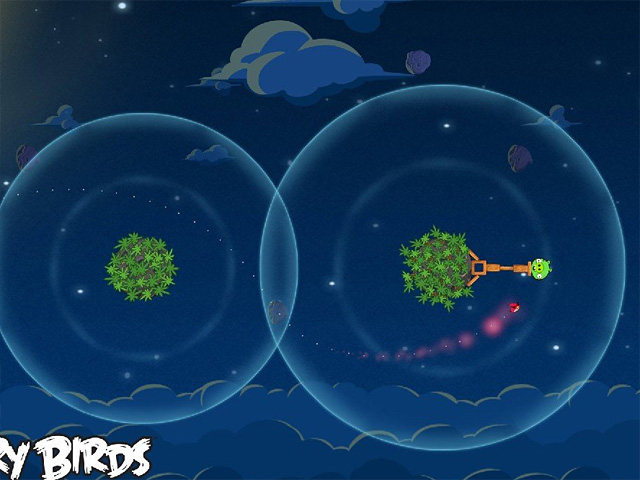# Simulate radial gravity (also known as “planet gravity”) with Box2D as seen on Angry Birds Space

With the launch of Angry Birds Space I am sure you are wondering how to simulate planet gravity with Box2D.And guess what… the basics are very easy.

First, in space there’s no gravity, so you will create a b2World world without gravity this way:

`private var world:b2World=new b2World(new b2Vec2(0,0),true);`

Then, it’s just a matter of applying Forces according to bodies and planets position.

Look at this script:

```package {
import flash.display.Sprite;
import flash.events.Event;
import flash.events.MouseEvent;
import Box2D.Dynamics.*;
import Box2D.Collision.*;
import Box2D.Collision.Shapes.*;
import Box2D.Common.Math.*;
import Box2D.Dynamics.Joints.*;
public class Main extends Sprite {
private var world:b2World=new b2World(new b2Vec2(0,0),true);
private var worldScale:Number=30;
private var planetVector:Vector.=new Vector.();
private var debrisVector:Vector.=new Vector.();
private var orbitCanvas:Sprite=new Sprite();
public function Main() {
orbitCanvas.graphics.lineStyle(1,0xff0000);
debugDraw();
}
private function createDebris(e:MouseEvent):void {
}
var fixtureDef:b2FixtureDef = new b2FixtureDef();
fixtureDef.restitution=0;
fixtureDef.density=1;
var circleShape:b2CircleShape=new b2CircleShape(r/worldScale);
fixtureDef.shape=circleShape;
var bodyDef:b2BodyDef=new b2BodyDef();
bodyDef.userData=new Sprite();
bodyDef.position.Set(pX/worldScale,pY/worldScale);
var thePlanet:b2Body=world.CreateBody(bodyDef);
planetVector.push(thePlanet);
thePlanet.CreateFixture(fixtureDef);
orbitCanvas.graphics.drawCircle(pX,pY,r*3);
}
var polygonShape:b2PolygonShape = new b2PolygonShape();
polygonShape.SetAsBox(w/worldScale/2,h/worldScale/2);
var fixtureDef:b2FixtureDef = new b2FixtureDef();
fixtureDef.density=1;
fixtureDef.friction=1;
fixtureDef.restitution=0;
fixtureDef.shape=polygonShape;
var bodyDef:b2BodyDef = new b2BodyDef();
bodyDef.type=b2Body.b2_dynamicBody;
bodyDef.position.Set(pX/worldScale,pY/worldScale);
var box:b2Body=world.CreateBody(bodyDef);
debrisVector.push(box);
box.CreateFixture(fixtureDef);
}
private function debugDraw():void {
var debugDraw:b2DebugDraw = new b2DebugDraw();
var debugSprite:Sprite = new Sprite();
debugDraw.SetSprite(debugSprite);
debugDraw.SetDrawScale(worldScale);
debugDraw.SetFlags(b2DebugDraw.e_shapeBit|b2DebugDraw.e_jointBit);
debugDraw.SetFillAlpha(0.5);
world.SetDebugDraw(debugDraw);
}
private function update(e:Event):void {
world.Step(1/30, 10, 10);
world.ClearForces();
for (var i:int=0; i
The whole code just create static bodies (planets) and let you place dynamic bodies (debris) with the click of the mouse.
The only interesting part of the script is the `for` loop in the `update` function, which I'll explain line by line:
for (var i:int=0; i
Loop which scans for all debris previously stored in `debrisVector` Vector declared at line 14 and updated at line 54
var debrisPosition:b2Vec2=debrisVector[i].GetWorldCenter();
Gets debris position
for (var j:int=0; j
Loop which scans for all planets previously stored in `planetVector` Vector declared at line 13 and updated at line 38
var planetShape:b2CircleShape=planetVector[j].GetFixtureList().GetShape() as b2CircleShape;
I need to know the mass of the planet because the bigger the mass, the more intense the gravity attraction. Unfortunately Box2D static bodies do not have mass, so I need to get the circle shape of the planet...
... and get its radius. So in this case the bigger the radius, the more intense the gravity attraction
var planetPosition:b2Vec2=planetVector[j].GetWorldCenter();
Gets planet position
var planetDistance:b2Vec2=new b2Vec2(0,0);
Creates a new b2Vec2 variable which will store the distance between the planet and the debris
planetDistance.Subtract(planetPosition);
... subtracts planet coordinates
var finalDistance:Number=planetDistance.Length();
Calculates the distance between the planet and the debris
Checks if the debris should be affected by planet gravity (in this case, the debris must be within a radius of three times the planet radius)
planetDistance.NegativeSelf();
Inverts planet distance, so that the force will move the debris in the direction of the planet origin
var vecSum:Number=Math.abs(planetDistance.x)+Math.abs(planetDistance.y);
Gets the sum of distance vector components. I will need this to make gravity attraction weaker when the debris is far from the planet, and stronger when the debris is getting close to the planet
This is the final formula to make the gravity weaker as we move far from the planet
debrisVector[i].ApplyForce(planetDistance,debrisVector[i].GetWorldCenter());
And finally the force can be applied to debris
This is the result:

Click to create debris.
```
214 GAME PROTOTYPES EXPLAINED WITH SOURCE CODE
// 1+2=3
// 10000000
// 2 Cars
// 2048
// Avoider
// Ballz
// Block it
// Blockage
// Bloons
// Boids
// Bombuzal
// Breakout
// Bricks
// Columns
// CubesOut
// Dots
// DROP'd
// Dudeski
// Eskiv
// Filler
// Fling
// Globe
// HookPod
// Hundreds
// InkTd
// Iromeku
// Lumines
// Magick
// MagOrMin
// Maze
// Memdot
// Nano War
// Nodes
// o:anquan
// Ononmin
// Pacco
// Phyballs
// Platform
// Poker
// Pool
// Poux
// Pudi
// qomp
// Racing
// Renju
// SameGame
// Security
// Sling
// Slingy
// Sokoban
// Splitter
// Sproing
// Stack
// Stringy
// Sudoku
// Tetris
// Threes
// Toony
// Turn
// TwinSpin
// vvvvvv
// Wordle
// Worms
// Yanga
// Zhed
// zNumbers